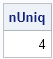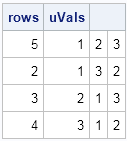How do you count the number of unique rows in a matrix? The simplest algorithm is to sort the data and then iterate down the rows, comparing each row with the previous row. However, this algorithm has two shortcomings: it physically sorts the data (which means that the original locations of the unique rows are lost) and the "iterate down the rows" phrase hints at using a loop over the rows. In a matrix language such as SAS/IML, R, or MATLAB, you should try to avoid unnecessary loops over rows of a matrix.

Fortunately, there is an easy way to count the number of unique rows of a matrix without physically sorting the matrix or writing any loops. The SAS/IML language provides the SORTNDX function, which computes a permutation of the rows that would sort the matrix. You can use the permutation vector in conjunction with the UNIQUEBY function, to count the number of unique rows in a matrix.

As an example, the following 6 x 3 matrix has only four unique rows. The first and fourth rows are the same, as are the third and sixth rows.

```proc iml; x = { 3 1 2, 1 3 2, 2 1 3, 3 1 2, /* duplicate of row 1 */ 1 2 3, 2 1 3 }; /* duplicate of row 3 */```

To find the number of unique rows, call the SORTNDX and UNIQUEBY functions, and then count the number of elements in the vector that the UNIQUEBY function returns, as follows:

```/* find number of unique rows */ cols = 1:ncol(x); /* sort by all columns */ call sortndx(ndx, x, cols); /* ndx = permutation of rows that sorts matrix */ uRows = uniqueby(x, cols, ndx); /* locate unique rows of sorted matrix */ nUniq = nrow(uRows); print nUniq;```If you intend to use this operation often, you might want to encapsulate it into a user-defined function, as follows:

```start NumUniqRows(x); cols = 1:ncol(x); call sortndx(ndx, x, cols); return( nrow(uniqueby(x, cols, ndx)) ); finish;```

Sometimes it is not sufficient to know the number of unique rows, you also want to know the values of the unique rows, and/or the locations of the rows in the original matrix, as follows:

```rows = ndx[uRows]; /* rows in original matrix */ uVals = x[rows, ]; /* values of unique rows */ print rows uVals;```Notice that the rows vector is not unique. For this example, the locations of the unique rows were found to be {5,2,3,4}. However, rows 1 and 4 are the same, as are rows 3 and 6. Therefore equivalent solutions include {5,2,6,4}, {5,2,3,1}, and {5,2,6,1}. Because the SORTNDX suroutine uses a sorting algorithm, it is difficult to predict which solution you will obtain until you actually run the algorithm. However, the unique values, which are returned in sorted order, are the same for each of these valid solutions.

If you will be finding the unique rows of a matrix (or equivalently, eliminating duplicate rows), you can create a SAS/IML module:

```/* get the unique rows in a matrix */ start GetUniqueRows(x); cols = 1:ncol(x); /* sort by all columns */ call sortndx(ndx, x, cols); /* ndx = permutation of rows that sorts matrix */ uRows = uniqueby(x, cols, ndx); /* locate unique rows of sorted matrix */ rows = ndx[uRows]; /* rows in original matrix */ return x[rows, ]; /* values of unique rows */ finish;```
Share

### About AuthorDistinguished Researcher in Computational Statistics

Rick Wicklin, PhD, is a distinguished researcher in computational statistics at SAS and is a principal developer of PROC IML and SAS/IML Studio. His areas of expertise include computational statistics, simulation, statistical graphics, and modern methods in statistical data analysis. Rick is author of the books Statistical Programming with SAS/IML Software and Simulating Data with SAS.

### 3 Comments

1.nice use of the sortndx function, I wasn't aware of it.. So for what known problems one would need to know the number of unique rows in a matrix?

•If you have transactional data, you might want to know how many unique customers there were.